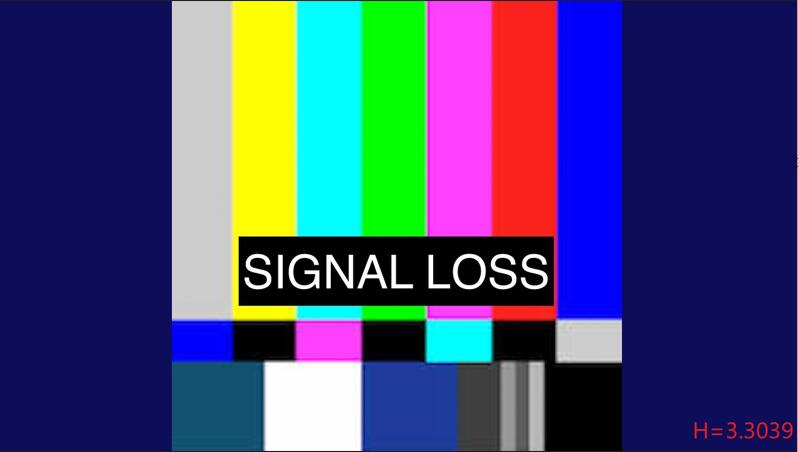## 1 图像二维熵

• 图像二维熵作为一种特征评价尺度能够反映出整个图像所含平均信息量的高低，熵值(H)越大则代表图像所包含的信息越多，反之熵值(H)越小，则图像包含的信息越少。对于图像信息量，可以简单地认为图像的边缘信息越多则图像的信息量越大。二维熵公式如下所示：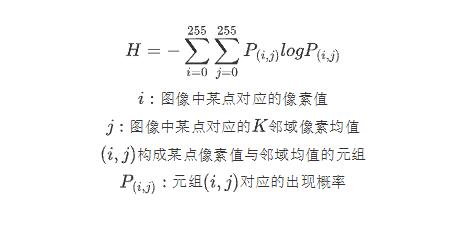## 2 信号丢失检测

### 2.1 画面对比

• 由于信号丢失所产生的画面大部分均由简单的纯色或少色的人造图像，再加上“信号丢失”提示信息所构成，因此信号丢失画面的信息量与正常图像相比较低，因此其对应的二维熵值更小。例如：上图所示的信号丢失画面由黑色屏幕加上信号丢失提示组成，画面简单，信息量较低

• 而正常画面具有更多的边缘信息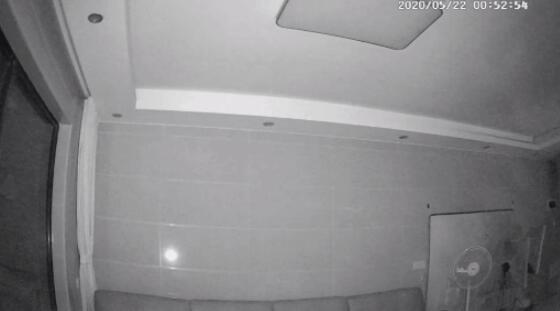相较于信号丢失画面，正常图像具有更高的信息量，其对应的二维熵值更大

### 2.2 基于图像二维熵的图像信号丢失检测C++实现(使用OpenCV)

• Step1：将输入的BGR图像转换为GRAY灰度图像。
Step2：计算图像中每个像素点对应地K(本文取K=8)邻域像素均值。
Step3：统计各（像素值，K邻域像素均值）二元组出现概率。
Step4：计算图像二维熵H。
Step5：将H与预设阈值Threshold进行比较，判断图像是否存在信号丢失异常。
``````bool SignalLossDetection::SignalEntropyLossException(cv::Mat& inputImg, double threshold)
{
//convert the input BGR image to GRAY iamge
cv::cvtColor(inputImg, inputImg, cv::COLOR_BGR2GRAY);
inputImg.convertTo(inputImg, CV_64F);
cv::Mat imgEntropyMap= cv::Mat::zeros(256, 256, CV_64F);// 256 * 256 entropy map
//calculate the mean value of K=8 neighborhood
cv::Mat meanKernal(3, 3, CV_16S);
short mean[]{ 1,1,1,
1,0,1,
1,1,1 };
meanKernal.data = (unsigned char*)mean;
cv::Mat meanMap;
cv::filter2D(inputImg, meanMap, -1, meanKernal, cv::Point(-1, -1), 0.0, cv::BORDER_REFLECT_101);
meanMap /= 8;

//calculate the (intensity, mean intensity of the K=8 neighborhood) two-tuples of the image
inputImg.convertTo(inputImg, CV_8UC1);
meanMap.convertTo(meanMap, CV_8UC1);
for(int i{0};i<meanMap.rows;++i)
for (int j{ 0 }; j < meanMap.cols; ++j) {
imgEntropyMap.at<double>(inputImg.at<uchar>(i, j), meanMap.at<uchar>(i, j))+=1;
}

//calculate the two dimensional entropy of the image
imgEntropyMap /= (inputImg.rows * inputImg.cols);
cv::Mat logMap;
cv::log(imgEntropyMap + 1e-7, logMap);//add delta=1e-7 to avoid overflow
imgEntropyMap = imgEntropyMap.mul(logMap);
if (-cv::sum(imgEntropyMap) <= threshold)//determine whether the image have the signal loss exception
return true;
else
return false;
}
``````

### 2.3 信号丢失检测结果分析

• 一张正常图像的二维熵值通常较高，如下图所示H=6.6348，说明图像中存在较多的边缘信息，不存在信号丢失现象。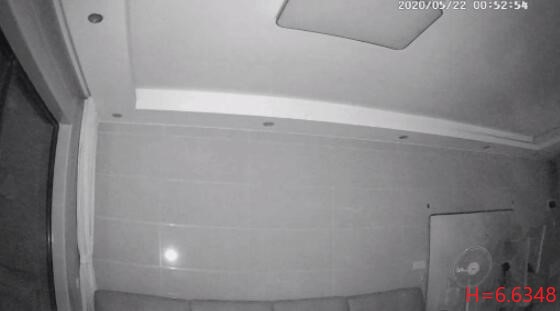• 作为最为常见的信号丢失情况，纯色背景的信号丢失图像的二维熵值极小，如下图所示H=0.3419，表明画面存在信号丢失异常。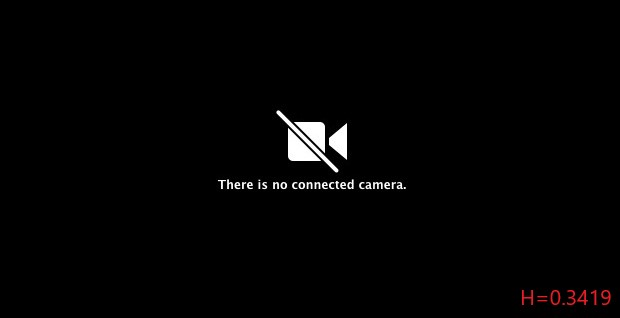• 彩色背景的信号丢失图像使用频率虽然不如纯色背景高，但也有大量的应用场景，通过计算它的二维熵值，发现其二维熵值虽然较纯色信号丢失图像更大，但依旧不高，如下图所示H=3.3039，依然可以判断图像存在信号丢失异常。